# Resources tagged with: Multiplication & division

Filter by: Content type:
Age range:
Challenge level:

### There are 135 results

Broad Topics > Calculations and Numerical Methods > Multiplication & division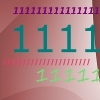### Ones Only

##### Age 11 to 14 Challenge Level:

Find the smallest whole number which, when mutiplied by 7, gives a product consisting entirely of ones.### Tom's Number

##### Age 7 to 11 Challenge Level:

Work out Tom's number from the answers he gives his friend. He will only answer 'yes' or 'no'.### What Two ...?

##### Age 7 to 11 Short Challenge Level:

56 406 is the product of two consecutive numbers. What are these two numbers?### Multiples Sudoku

##### Age 11 to 14 Challenge Level:

Each clue in this Sudoku is the product of the two numbers in adjacent cells.### Divide it Out

##### Age 7 to 11 Challenge Level:

What is the lowest number which always leaves a remainder of 1 when divided by each of the numbers from 2 to 10?### Difficulties with Division

##### Age 5 to 11

This article for teachers looks at how teachers can use problems from the NRICH site to help them teach division.### Ducking and Dividing

##### Age 7 to 11 Challenge Level:

Your vessel, the Starship Diophantus, has become damaged in deep space. Can you use your knowledge of times tables and some lightning reflexes to survive?### Escape from the Castle

##### Age 7 to 11 Challenge Level:

Skippy and Anna are locked in a room in a large castle. The key to that room, and all the other rooms, is a number. The numbers are locked away in a problem. Can you help them to get out?### Being Resilient - Primary Number

##### Age 5 to 11 Challenge Level:

Number problems at primary level that may require resilience.### Learning Times Tables

##### Age 5 to 11 Challenge Level:

In November, Liz was interviewed for an article on a parents' website about learning times tables. Read the article here.### What Is Ziffle?

##### Age 7 to 11 Challenge Level:

Can you work out what a ziffle is on the planet Zargon?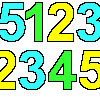### 1, 2, 3, 4, 5

##### Age 7 to 11 Challenge Level:

Using the numbers 1, 2, 3, 4 and 5 once and only once, and the operations x and ÷ once and only once, what is the smallest whole number you can make?### Surprising Split

##### Age 7 to 11 Challenge Level:

Does this 'trick' for calculating multiples of 11 always work? Why or why not?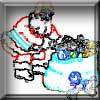### Clever Santa

##### Age 7 to 11 Challenge Level:

All the girls would like a puzzle each for Christmas and all the boys would like a book each. Solve the riddle to find out how many puzzles and books Santa left.### Domino Numbers

##### Age 7 to 11 Challenge Level:

Can you see why 2 by 2 could be 5? Can you predict what 2 by 10 will be?### Oh! Hidden Inside?

##### Age 11 to 14 Challenge Level:

Find the number which has 8 divisors, such that the product of the divisors is 331776.### Repeaters

##### Age 11 to 14 Challenge Level:

Choose any 3 digits and make a 6 digit number by repeating the 3 digits in the same order (e.g. 594594). Explain why whatever digits you choose the number will always be divisible by 7, 11 and 13.### Amy's Dominoes

##### Age 7 to 11 Challenge Level:

Amy has a box containing domino pieces but she does not think it is a complete set. She has 24 dominoes in her box and there are 125 spots on them altogether. Which of her domino pieces are missing?### Eminit

##### Age 11 to 14 Challenge Level:

The number 8888...88M9999...99 is divisible by 7 and it starts with the digit 8 repeated 50 times and ends with the digit 9 repeated 50 times. What is the value of the digit M?### Book Codes

##### Age 7 to 11 Challenge Level:

Look on the back of any modern book and you will find an ISBN code. Take this code and calculate this sum in the way shown. Can you see what the answers always have in common?### Magic Potting Sheds

##### Age 11 to 14 Challenge Level:

Mr McGregor has a magic potting shed. Overnight, the number of plants in it doubles. He'd like to put the same number of plants in each of three gardens, planting one garden each day. Can he do it?##### Age 11 to 14 Challenge Level:

Visitors to Earth from the distant planet of Zub-Zorna were amazed when they found out that when the digits in this multiplication were reversed, the answer was the same! Find a way to explain. . . .### X Marks the Spot

##### Age 11 to 14 Challenge Level:

When the number x 1 x x x is multiplied by 417 this gives the answer 9 x x x 0 5 7. Find the missing digits, each of which is represented by an "x" .### One O Five

##### Age 11 to 14 Challenge Level:

You can work out the number someone else is thinking of as follows. Ask a friend to think of any natural number less than 100. Then ask them to tell you the remainders when this number is divided by. . . .### One Million to Seven

##### Age 7 to 11 Challenge Level:

Start by putting one million (1 000 000) into the display of your calculator. Can you reduce this to 7 using just the 7 key and add, subtract, multiply, divide and equals as many times as you like?### A First Product Sudoku

##### Age 11 to 14 Challenge Level:

Given the products of adjacent cells, can you complete this Sudoku?### Multiplication Series: Illustrating Number Properties with Arrays

##### Age 5 to 11

This article for teachers describes how modelling number properties involving multiplication using an array of objects not only allows children to represent their thinking with concrete materials,. . . .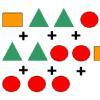### Super Shapes

##### Age 7 to 11 Short Challenge Level:

The value of the circle changes in each of the following problems. Can you discover its value in each problem?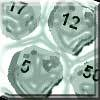### Asteroid Blast

##### Age 7 to 11 Challenge Level:

A game for 2 people. Use your skills of addition, subtraction, multiplication and division to blast the asteroids.### Odds and Threes

##### Age 7 to 11 Challenge Level:

A game for 2 people using a pack of cards Turn over 2 cards and try to make an odd number or a multiple of 3.### More Magic Potting Sheds

##### Age 11 to 14 Challenge Level:

The number of plants in Mr McGregor's magic potting shed increases overnight. He'd like to put the same number of plants in each of his gardens, planting one garden each day. How can he do it?### Two Many

##### Age 11 to 14 Challenge Level:

What is the least square number which commences with six two's?### 3388

##### Age 11 to 14 Challenge Level:

Using some or all of the operations of addition, subtraction, multiplication and division and using the digits 3, 3, 8 and 8 each once and only once make an expression equal to 24.##### Age 11 to 14 Challenge Level:

Powers of numbers behave in surprising ways. Take a look at some of these and try to explain why they are true.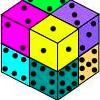### Six Numbered Cubes

##### Age 7 to 11 Challenge Level: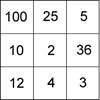### Four Go for Two

##### Age 7 to 11 Challenge Level:

Four Go game for an adult and child. Will you be the first to have four numbers in a row on the number line?### Square Subtraction

##### Age 7 to 11 Challenge Level:

Look at what happens when you take a number, square it and subtract your answer. What kind of number do you get? Can you prove it?### Looking at Lego

##### Age 7 to 11 Challenge Level:

This task offers an opportunity to explore all sorts of number relationships, but particularly multiplication.### Remainders

##### Age 7 to 14 Challenge Level:

I'm thinking of a number. My number is both a multiple of 5 and a multiple of 6. What could my number be?### The Clockmaker's Birthday Cake

##### Age 7 to 11 Challenge Level:

The clockmaker's wife cut up his birthday cake to look like a clock face. Can you work out who received each piece?### Sort Them Out (2)

##### Age 7 to 11 Challenge Level:

Can you each work out the number on your card? What do you notice? How could you sort the cards?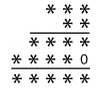### Long Multiplication

##### Age 11 to 14 Challenge Level:

A 3 digit number is multiplied by a 2 digit number and the calculation is written out as shown with a digit in place of each of the *'s. Complete the whole multiplication sum.##### Age 11 to 14 Challenge Level:

If you take a three by three square on a 1-10 addition square and multiply the diagonally opposite numbers together, what is the difference between these products. Why?### Like Powers

##### Age 11 to 14 Challenge Level:

Investigate $1^n + 19^n + 20^n + 51^n + 57^n + 80^n + 82^n$ and $2^n + 12^n + 31^n + 40^n + 69^n + 71^n + 85^n$ for different values of n.### Countdown

##### Age 7 to 14 Challenge Level:

Here is a chance to play a version of the classic Countdown Game.### Napier's Bones

##### Age 7 to 11 Challenge Level:

The Scot, John Napier, invented these strips about 400 years ago to help calculate multiplication and division. Can you work out how to use Napier's bones to find the answer to these multiplications?### The Remainders Game

##### Age 7 to 14 Challenge Level:

Play this game and see if you can figure out the computer's chosen number.### Number Tracks

##### Age 7 to 11 Challenge Level:

Ben’s class were cutting up number tracks. First they cut them into twos and added up the numbers on each piece. What patterns could they see?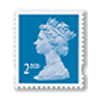### Penta Post

##### Age 7 to 11 Challenge Level:

Here are the prices for 1st and 2nd class mail within the UK. You have an unlimited number of each of these stamps. Which stamps would you need to post a parcel weighing 825g?### The Pied Piper of Hamelin

##### Age 7 to 11 Challenge Level:

This problem is based on the story of the Pied Piper of Hamelin. Investigate the different numbers of people and rats there could have been if you know how many legs there are altogether!